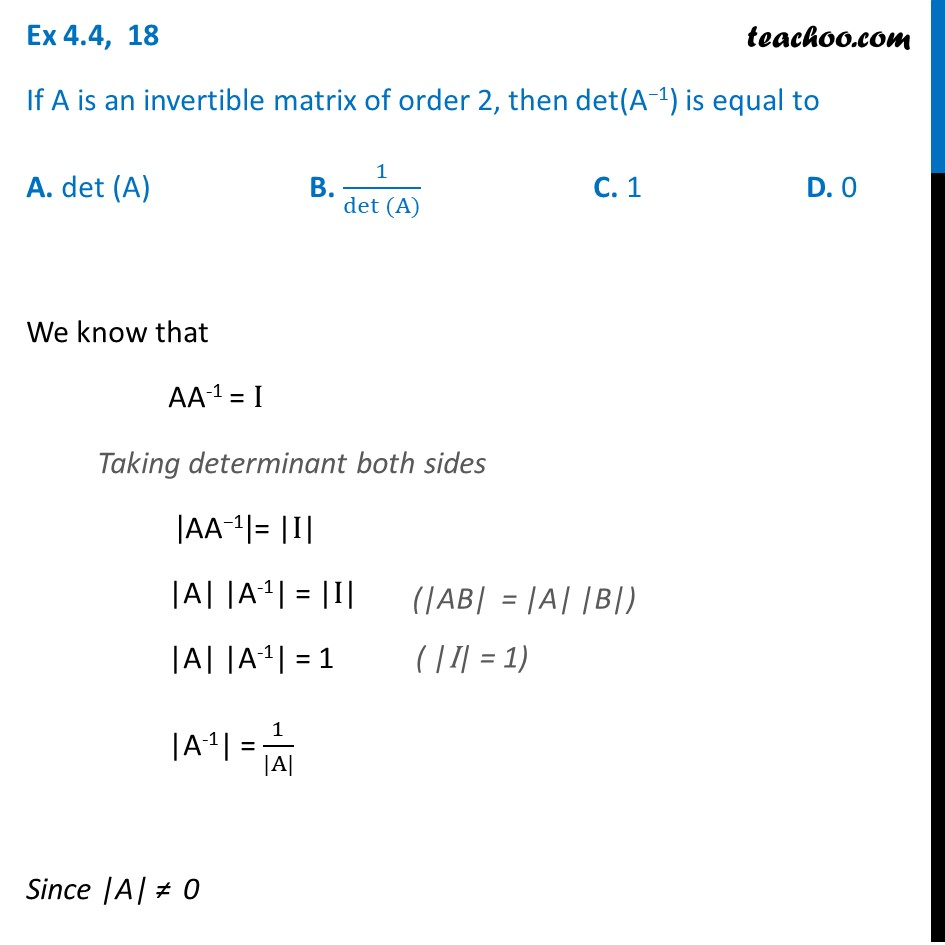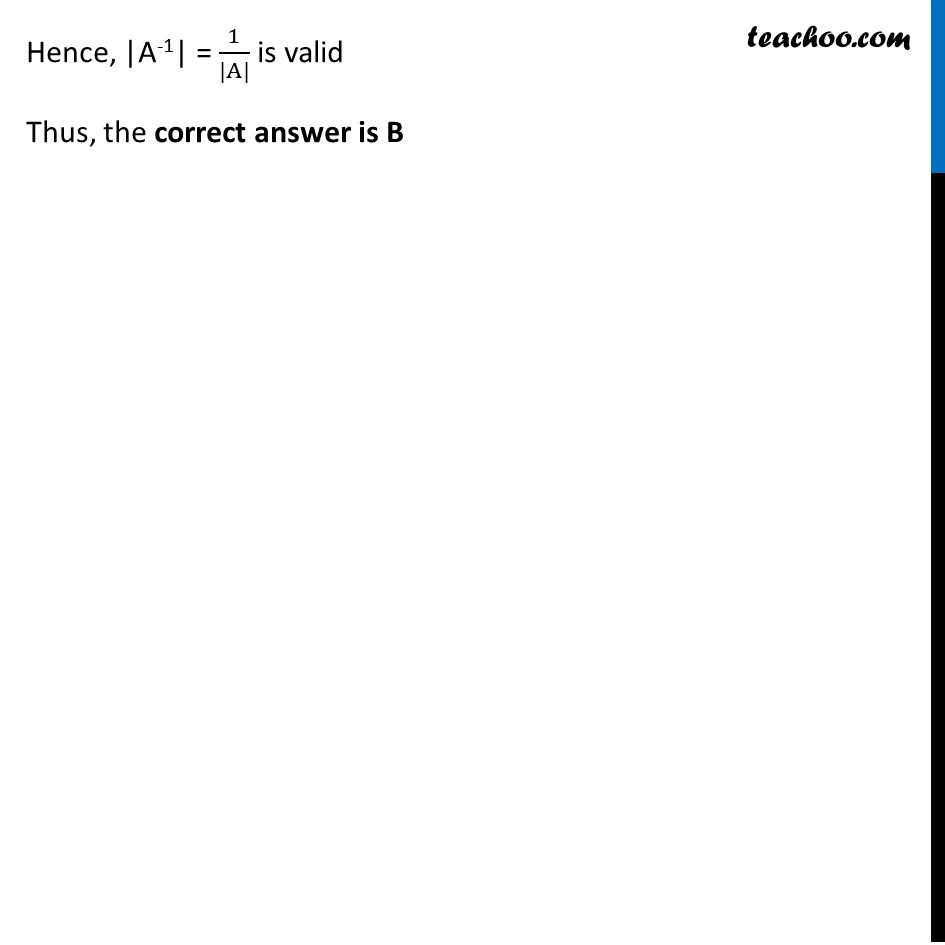Ex 4.4

Chapter 4 Class 12 Determinants
Serial order wiseLearn in your speed, with individual attention - Teachoo Maths 1-on-1 Class

### Transcript

Ex 4.4, 18 If A is an invertible matrix of order 2, then det(A−1) is equal to A. det (A) B. 1/(det (A)) C. 1 D. 0 We know that AA-1 = I Taking determinant both sides |"AA−1" |= |I| |A| |A-1| = |I| |A| |A-1| = 1 |A-1| = 1/(|A|) Since |A| ≠ 0 Hence, |A-1| = 1/(|A|) is valid Thus, the correct answer is B